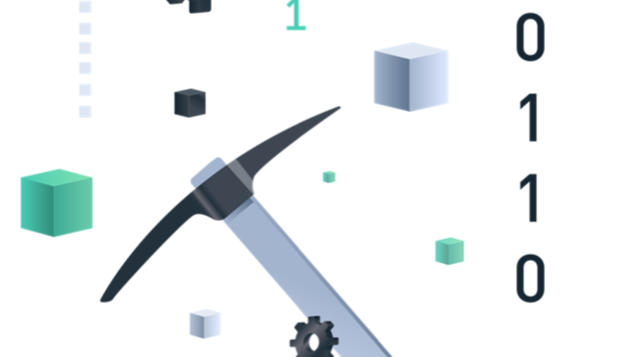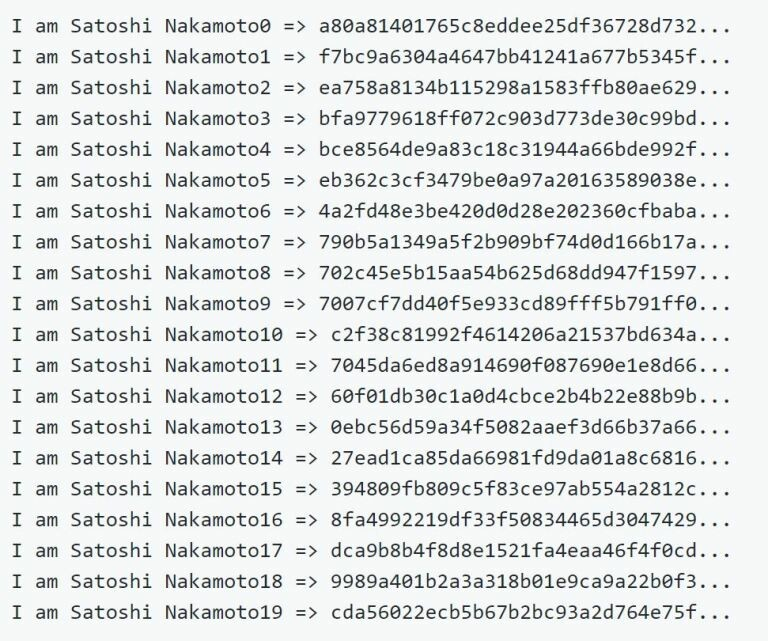Proof-of-Work - the algorithm that makes Bitcoin move# Proof-of-Work - the algorithm that makes Bitcoin move

By Simplify Crypto | Simplify Crypto | 15 Sep 2020

To understand the role of miners and how consensus is achieved through a decentralized network with thousands of nodes without a central authority, we must first understand the innovative algorithm that makes all of this possible.

A hash algorithm turns a data input of arbitrary size into a deterministic result of defined size, a fingerprint of the input. For a specific input the resulting hash will always be the same and can be easily verified and validated by whoever runs the same algorithm. The vital characteristics of a computational hash algorithm are that it is computationally infeasible to discover two different inputs that produce the same result and that it is practically impossible to select the input that will give the desired result, other than trying random inputs. We refer to the results as fingerprints because they are unique.

In the Bitcoin network, miners use the SHA256 cryptographic algorithm. A Secure Hash Algorithm (AHS) that transforms any data that is entered into an output with a fixed size of 256 bits. In its simplest form, what miners do is run the header of a validated block repeatedly, changing a single parameter until the result is less than a well-defined target. This result cannot be determined at the outset, nor can a standard be defined to produce a specific result. Since the only way to achieve the result is through random variation, it is said that computational brute force is used.

The way the SHA256 works, any tiny change in the input will significantly change the final result. Just a letter, a punctuation mark or any other character will produce (with an enormous probability) a totally different result.The variable used as a parameter to be changed is called a nonce. By varying the nonce used, the result of the cryptographic algorithm is varied until the desired value is found. That is, the function will vary the output until it has a result lower than that defined by the target. The limit defined by the algorithm is known as a target and will be inversely proportional to the difficulty in finding a result that satisfies this condition - being lower than the target. The smaller the target, the greater the difficulty.

A good example to understand this concept is a craps game where players have to roll the dice with less than a predefined target. If we say the target is 12, a player will only lose if he rolls six on each of the dice. However, if we decrease the target, the number of combinations that will cause a player to lose will increase. For example, if we set the target to 5, more than half of the combinations will not serve to win the game. On average, players will have to launch exponentially more times each time the target is lowered. When the target is 2, only one combination in 36 will be the winning combination, only 2% of the possibilities. An outside observer who knows that the target is 2, can assume that a winner launched an average of 36 times. That is, it is possible to estimate the work invested to win, based on the difficulty imposed by the target.

##### In the SHA256 algorithm, the presentation of the input itself - which will create the desired result - serves as proof of the work done to achieve the result below the defined target. Therefore, the algorithm used in the Bitcoin network is called Proof-of-Work.

Despite the large amount of computational brute force work needed to find the result below the target, it is necessary to run the function only once to verify that it uses the correct variable that produces that result. Therefore, after a miner sends the result, all nodes in the network will be able to verify that he found the correct result and spent the necessary work to achieve it. Upon accepting this result the block will be published on the blockchain and the miner will be entitled to the reward of the bitcoins created in that block.

As we have seen, the difficulty in discovering the target forces multiple hashes to be attempted until it is possible to discover the result that satisfies the function and assigns the reward. In the Bitcoin network this “game” is a competition to publish the next block on the network and to be able to receive the bitcoins, included in that block. If these become more attractive, more miners will join the network. The network gets stronger as there are more miners, which means that there is more computational power to reach the target. The difficulty is calculated for the total power of the network, thus, increasing the power of the network, the result would be found more quickly and the consistency of 10 minutes per block would be lost.

To avoid losing this characteristic, the network adjusts the target by itself and, thus, also adjusts the difficulty in finding the result and manages to maintain the publication of a block every 10 minutes. The target adjustment is made every 2016 blocks. The algorithm measures the time it took to discover the last 2016 blocks and divides it by 20160 minutes (the optimal time to publish that number of blocks by publishing one block every 10 minutes). By calculating the ratio between real time and desired time, adjust the difficulty by increasing or decreasing it as necessary.

##### Simply put: if the network finds blocks in less than 10 minutes, the difficulty increases, decreasing the target. If the network discovers blocks, on average, in more than 10 minutes, the difficulty decreases. To do this, increase the target.

In order to control the volatility in the variation of the difficulty, the target adjustment will always be less than a factor of 4 per cycle of 2016 blocks (about two weeks). If the difficulty has to be increased significantly, it will be increased to a maximum of a factor of 4. The necessary adjustments will be made in the next cycle.

Note that the target is independent of the number or value of transactions. The increase in the total computational power of the network represents the entry of new miners into the competition for the reward. The relationship between the price of electricity to power a mining computer and the value of Bitcoin will be the determining factor for the entry of new miners as this is the determining factor in the incentives to enter or leave the market, since each miner is free from do it every time you want.Simplify Crypto

Trying to help people to understand the crypto world from the basic concepts to the crypto projects purpose. My articles are publish in Publish0x and HiveSend a \$0.01 microtip in crypto to the author, and earn yourself as you read!

20% to author / 80% to me.
We pay the tips from our rewards pool.